Courses

# Design Of Packed Tower Chemical Engineering Notes | EduRev

## Chemical Engineering : Design Of Packed Tower Chemical Engineering Notes | EduRev

The document Design Of Packed Tower Chemical Engineering Notes | EduRev is a part of the Chemical Engineering Course Mass Transfer.
All you need of Chemical Engineering at this link: Chemical Engineering

Design of packed tower
The cross sectional view of the packed tower is shown in Figure 4.5.

Design of packed tower may be
(I) on the basis of individual mass transfer coefficients or
(II) on the basis of overall mass transfer coefficient.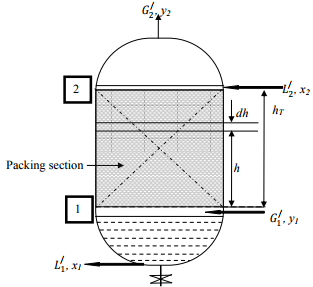Figure 4.5: Cross sectional view of packed tower.

The column is packed with packing materials (any type) to provide more contact between gas and liquid.
Let, Gand L/ are gas and liquid flow rate per unit area basis, mol/h.m2. ā is specific interfacial contact area between gas and liquid, m2 /m3 . The mole fraction of solute in gas is y. Hence, solute flow rate in gas= G/y mol/h.m2
The decrease in solute flow rate over the thickness dh=d(G/y)                                                                                                   (4.1)
For a unit cross-sectional area (1m2 ), volume of differential section=1×dh m3 and interfacial area of contact in differential section= ā×1×dh m2
If NA is solute flux and ky is individual gas-phase mass transfer coefficient, solute transfer through differential section= ā×dh×NA.
Therefore,
-[G/dy+ y dG/]= ā×dh×NA                                                                       (4.2)
-G/dy - y dG/ = ā×dh×NA                                                                        (4.3)
The change in total gas flow rate (dG) is equal to rate of solute transfer (ā×dh×NA) as carrier gas is not soluble, i.e.,
- dG= ā×dh×NA                                                                                   (4.4)
Putting the value of –dG/ in Equation 4.3, we have,
-G/dy + ā×dh×NA y= ā×dh×NA                                                            (4.5)
-G/dy = ā×dh×NA(1-y)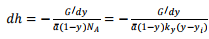(4.6)
Boundary conditions:
h=0; y=y1
h=hT; y=y
Integration of Equation 4.6 gives the height of packed column as follows: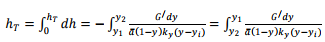(4.7)
Interfacial solute concentration, yi is not known; hence the integration of the right hand side of Equation 4.7 is complicated.

STEP-BY-STEP PROCEDURE
(1) For a particular gas-liquid system, draw equilibrium curve on X-Y plane.
(2) Draw operating line in X-Y plane (PQ) using material balance Equation.
Lower terminal Q (X2, Y2) and upper terminal P (X1, Y1) are placed in x-y plane. Overall mass balance Equation for the absorption tower is as follows: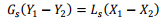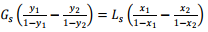(4.8)
If liquid mass flow rate, Ls is not known, minimum liquid mass flow rate (Ls)min is to be determined. Ls is generally 1.2 to 2 times the (Ls)min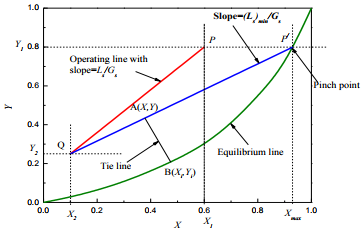Figure 4.6: Graphical determination of (Ls)min for absorption.

In Figure 4.6, lower terminal of absorption tower is represented by Q (X2, Y2); i.e., bottom of the tower. Operating line is PQ. If liquid rate is decreased, slope of operating line (Ls/Gs) also decreases and operating line shifts from PQ to P/Q, when touches equilibrium line. This operating line is tangent to equilibrium line.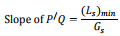The driving force for absorption is zero at P/ and is called “PINCH POINT”.

(3) A point A (x, y) is taken on the operating line. From the known value of kx and ky or kxā and kyā, a line is drawn with slope of kx/ky to equilibrium line, B(xi,yi). Line AB is called “TIE LINE” and xi and yi are known for a set of values of x and y.
(4) Step (3) is repeated for other points in the operating line to get several (xi,yi) sets for y1≥y≥y2.
(5) Calculate flow rate of gas G (kg/h) at each point as G=Gs(1+y).
(6) Calculate height of the packing hT of Equation 4.7 graphically or numerically.
The height of the „stripping column‟ is also obtained in a similar way. For stripping, y2>y1 and driving force is (yi-y). The corresponding design Equation will be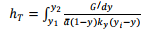(4.9)

Offer running on EduRev: Apply code STAYHOME200 to get INR 200 off on our premium plan EduRev Infinity!

## Mass Transfer

29 videos|46 docs|44 tests

,

,

,

,

,

,

,

,

,

,

,

,

,

,

,

,

,

,

,

,

,

;